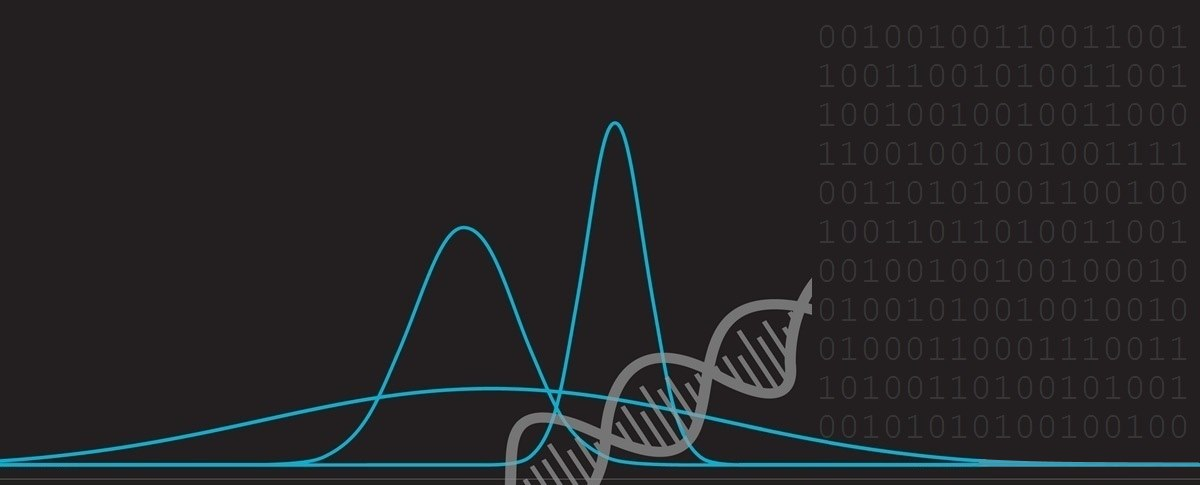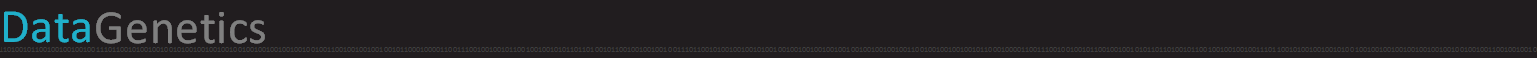# Yahtzee Probability

 Santa brought my kids the game of Yahtzee for Christmas. We’ve been playing it a lot in the evenings. When a Yahtzee is rolled, my kids go wild. In this blog posting I'm going to look into the probability of rolling a Yahtzee.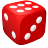Yahtzee is a game played with five six-sided dice. A player rolls the dice, examines the results and can keep as many of the dice as they like, re-rolling the remainder. After the second roll, the process is repeated (if desired, the player can pick-up dice held on the first round). After (up-to) three rolls, the dice are scored according to various categories. The Yahtzee (scoring 50 points) is achieved by getting all five dice the same.

### Assumptions

We’re going to assume the player is a smart player, and at each decision point makes the smartest possible re-roll and hold choices.

The probability of getting a Yahtzee in a single roll is easy to calculate. There are five dice, so whatever the first die rolls there is a 1/6 chance that the second die is the same number. If that occurs, there’s a 1/6 chance that the third die is the same, ditto the fourth and the fifth.

So probability of Yahztee in one roll is 1/6 x 1/6 x 1/6 x 1/6 = 1/1296.

With three rolls and holding, however, things get a little more complicated. The number of dice we roll each turn can be changed, and there are many possible combinations to consider. Because the state of dice at the start of each roll is independent of how the dice were rolled, this is a perfect chance to roll out one of my favorite tools, the Markov Chain (for more background on this, see my earlier postings on CandyLand, and Chutes and Ladders).

# Combinatronics

 Before delving in the Markov Chain, however, it will benefit us to examine the various ways that combinations of dice can be rolled. This exercise will greatly simplify the creation of the Transition Matrix (trust me on this). Here we go …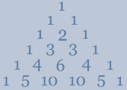## 2 diceThis is the trivial case. There are only two patterns for the way that two dice can be rolled. They either match, or they do not. There is a 1/6 chance that the second of the two dice will match the first, and conversely, there is a 5/6 chance that it will not match. The probabilities, of course, have to add up to 1 (one of the two events must occur).

Another way to look at this is that there are 36 possible combinations for two dice to be rolled. In six of these combinations {1.1}, {2,2}, {3,3}, {4,4}, {5,5}, {6,6} the numbers are the same, and in 30 of these combinations, the dice are different.These results are show graphically on the image above. There is a 6/36 chance that both dice will be the same, represented as {A,A}, and 30/36 chance they will be different, represented as {A,B}

## 3 diceThis gets a little more complex, but not much. Here there are three possible flavors of result for the three dice: They are either all the same, they are all different, or there will be two of one number and one of another. There are 216 possible ways that three dice can be rolled (6 x 6 x 6).

We can calculate the probability of them all being the same, easily, as 1/6 x 1/6 = 1/36 (The second die matches the first 1 time in 6, and the third die matches, again, 1 time in 6).

Alternatively, we can imagine that, of the 216 possible ways the dice could land, there are six possible ways when they are all the same: {1,1,1}, {2,2,2}, {3,3,3}, {4,4,4}, {5,5,5}, {6,6,6}.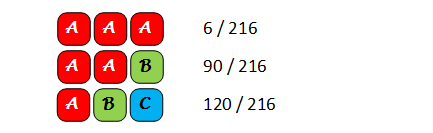The probability of them all be being different can be calculated using the following logic: The first die can be whatever it likes, then for the second die there is a 5/6 chance that it will not be the same number as the first die. Finally, there is a 4/6 chance that the third die will be different for the first two. So, the probability that all the dice will be different is 5/6 x 4/6 = 20/36 which can be unsimplified to 120/216. There are 120 possible combinations of the 216 possible outcomes where all three dice are different {A,B,C}.

Because we know that total of all probabilities for the way three dice roll must add up to 1.0 we can deduce that the probability of two of the dice being the same {A,A,B} is 90/216 (Which is 16/216120/216).

(If you want to convince yourself of this, think of it this way: There are six possible values that A could be, five possible values of what B could be, and three possible choices of which die could be B. This is 6 x 5 x 3 = 90 combinations out of the 216).

## 4 diceThings are starting to get a little more complex now. They can be all the same, all different, three of a kind, two lots of two pairs, or a pair with two different singletons.

There are 1296 ways that four dice can be arranged (6 x 6 x 6 x 6). The results are shown below:We need to be extra careful here, because we don’t want to double count. When counting the two lots of two pairs, we need to make sure that we don’t inadvertently count {5,5,5,5} as two sets of double five and place this in the {A,A,B,B} bucket, because it is really four of a kind and needs to be in the {A,A,A,A} bucket. If we double count, the probabilities will become greater than 1.0!

Deriving the above table can be done in a variety of ways. Those of you who studied math at university might reach for a binomial expansion for calculating the permutations. Alternatively, like we discussed in the Risk analysis page, the number of combinations is so small (just 1296) that you might want to simply brute-force all the combinations in code and count them.

It’s actually a good mental exercise to work through derivation of these numbers to convince yourself that the numbers are correct. For instance {A,A,A,A} is 1/6 x 1/6 x 1/6 for the chances that the second, third and four die match the first. (Alternative thinking, is that there are only six ways to get four of a kind = 6/1296).

For three of a kind {A,A,A,B} there are six possible numbers that A could be, and five possible numbers that B could be, and four locations for the dice B, which is 6 x 5 x 4 combinations = 120/1296.

For all the dice to be different {A,B,C,D} there is a 5/6 chance that the second dice is different from the first, and a 4/6 that the third is unique, and 3/6 chance that fourth is. 5/6 x 4/6 x 3/6 = 60/216 = 360/1296.

Interesting Note – As an aside here, when rolling four dice, the most likely outcome is that you will get a pair, and there is a greater than 72.2% chance that you will get a least a pair (720+90+120+6)/1296

## 5 diceNow things are getting a little busy! There are 7776 possible combinations for five dice. The results are shown below. In the interests of brevity, I’m not going to derive them all here (maybe in a future blog post), and I’ll simply show the results so we can get back to the Markov Chain.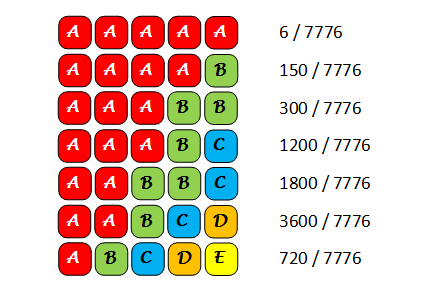Interesting Note – The probability of rolling a FullHouse in one roll is 300/7776 cf. Four of a kind at just 150/7776. According to our rules, a full house scores 25 points and (at most), the four of a kind can score 30 points (all sixes – Yahtzee bonus excluded), so the full house is an easy point pickup, being twice as easy as getting as four of a kind!

Interesting Note – With five dice, there is 7056/7776 chance that you will get a pair or better on your first roll (90.7%)

### Back to Markov

To perform our Markov analysis, we need to create a Transition Matrix which defines the probability of moving between each state.

As the states, I’m going to select the number of matching dice in the set, so we have 5 states: 1,2,3,4,5 (Here, "1" matching dice could also be described as a singleton). This results in a matrix of 25 elements.Our matrix will be upper triangular in nature (we made the assumption of a smart player thus, if there is a roll of three of a kind, we’re not going to suggest that the player re-rolls part of this in order to get to a Yahtzee!). The Transition Matrix will show the probabilities of moving from any state to either the same state, or a higher one. Here's the Transition Matrix. We need to populate each location containing a '?' with the probability of moving from between the state represented by the column number to the state represented by the row number. (All other locations have zero probability). Here we go …The first few entries are easy to fill in. The probability of moving from state 5 to state 5 is 1.0 Once we achieve a Yahtzee, we're going to keep it and not roll any more dice, so there is 100% certainty we'll keep in this state! If we currently have 4 matching dice, there is a 1/6 chance that we'll roll the correct number to make it 5, and correspondingly a 5/6 of staying in state 4. The stochastic nature of the transition matrix is maintained because the row of this matrix sums up to 1.0 (Something has to happen, and it will be one of these state changes).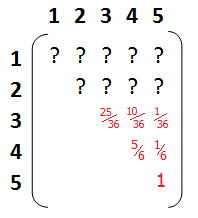For state 3, there are two dice to re-roll, giving 36 possible combinations. (Recall back to the section of combinatronics above). There is a 1/36 chance that both dice will match the current three of a kind to make a Yahtzee and this probability is placed in row 3 and column 5. There is a 25/36 chance that the player will still have three of a kind at the end of the next roll (5/6 chance of missing with the first die multiplied by 5/6 chance of missing with the second). Finally, there is a 10/36 chance of getting one additional number to make four of a kind. This is 1/6 x 5/6, and this can be achieved two different ways (either the first die matches, or the second one does).Things are getting a little trickier now, so we’ll slow down. Going from state 2 to state 5 requires all three re-rolled dice matching the current pair. This occurs with probability 1/216 (Which is 1/6 x 1/6 x 1/6). Similarly going from nothing matching, (state 1), to state 5, is the equivalent of rolling a Yahtzee in one go (because all the dice are re-rolled). This is 1/1296, calculated as (1/6 x 1/6 x 1/6 x 1/6).We can fill in two more coefficients. If you’re unlucky, and have nothing matching (state 1), and re-roll all the dice, the probability of getting nothing matching again is 120/1296 (This is 5/6 chance of the second die not matching, followed by 4/6 for the third and 3/6 of the fourth, and 2/6 for the fifth). The chances of rolling four of a kind in a single roll are 25/1296 (Which is 150/7776. Remember back to the Combinatronics section? It’s calculated by getting four dice the same: 1/6 x 1/6 x 1/6, with the last die not matching 5/6, and there are five possible ways this can be formed with the five possible locations for B in the set {A,A,A,A,B}. If it's not clearly obvious, the probabilities on the top row (changing from nothing matching to any other state), are the probabilities for the outcome of the first roll.The probability of getting three of a kind in one roll is 250/1296. This is a little more challenging to calculate, and we have to be careful. This occurs in one of two patterns {A,A,A,B,B} and {A,A,A,B,C}. Referencing the combinatronics section above (remember, I said it would be useful?), we can see that {A,A,A,B,B} occurs 300/7776 times (the full house) and {A,A,A,B,C} (three of a kind) occurs 1200/7776. Adding these together (the probabilistic way of saying OR) we get 1500/7776, which reduces to 250/1296. To fill in the last element in this row (getting a pair), again we have to be careful. There are two sets to consider: {A,A,B,C,D} and {A,A,B,B,C}. (Single Pair and Two Pair). Since we’re going for a Yahtzee, we roll the one of the pairs along with the singleton. The probability of getting a pair is 1800/7776 + 3600/7776 = 5400/7776 or 900/1296). It’s also relieving to know that all the probabilities in this row add up to 1.0 (120/1296 + 900/1296 + 250/1296 + 25/1296 +1/1296).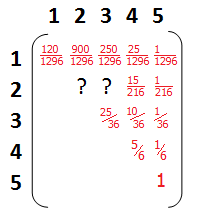Transitioning from a pair (state 2) to four of a kind (state 4) is probability 15/216. There is a 1/6 chance that one of the dies will match, then another 1/6 chance that another one will, multiplied by the 5/6 chance that the final die does not match. There are three possible ways for which of the 5/6 dice is, so the final probability = 3 x 1/6 x 1/6 x 5/6 = 15/216.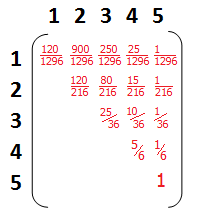Now the last two tricky ones. Reading a little on the internet, this is where people appear to slip up in their calculations. The complication arises because, when re-rolling three dice, you might want to "jump ship" about what you are aiming for. For example, if you rolled a pair on your first roll, kept the pair and re-rolled three dice, and these three dice all came up the same (but not the same as the pair, otherwise that would be Yahtzee!), then on the next roll you would want to keep the three of a kind and re-roll the pair. This subtlety modifies the probabilities. Let’s work through this – We’ve kept a pair and re-rolled three dice. Recall from our three dice combinatronics that three dice turn up all the same with probability 1/6 x 1/6 = 1/36 = 6/216, but in one of these cases, this number rolled will be the same as the pair (causing a Yahtzee), so we need to subtract this case. Thus, the chance of converting from two of a kind to three of a kind is 6/216 – 1/216 = 5/216.

To complete the coefficient of transition from a pair (state 2) to stay as a pair (state 2), we need the basic probability of this, which is simply 5/6 x 5/6 x 5/6 (all three dice miss matching the pair), and from this, we need to subtract the chance that all these three dice are the same and do not form a Yahtzee (5/216), so the final result for this element = 125/2165/216 = 120/216.

Similarly, the inverse of this trick for the transition probability from a pair state 2 to three of a kind state 3. Here basic probability of moving from state 2 to state 3 is 75/216 (three dice are rolled, with 1/6 chance of a match, then two 5/6 chances of a miss, and there are three combinations of ways this can be achieved which is 3 x 1/6 x 5/6 x 5/6). To this basic probability of 75/216, we need to add the 5/216 chance from above that we got to three of a kind through this alternate route.

Thus, the final element required to complete our Transition Matrix from state 2 to state 3 is 75/216 + 5/216 = 80/216.

It’s also a relief to calculate that the probabilities in this row total 1.0! (120/216 + 80/216 + 15/216 + 1/216). This helps confirm our calculations are correct.

Our Transition Matrix is now complete!

### Matrix Multiplication

We now input an identity column vector and multiply this by the Transition Matrix. The resultant row vector output shows the probabilities of the distrubutution of states that the dice could be in. We can now take this output and use it at as the input again and this time the output is the superposition of the probabilities for the dice at the end of the second roll. To get the probability of obtaining a Yahtzee on (or before) three rolls, we multiple one last time and read the result of the firth element (state 5) in the final output vector.

(From this point out, I'm switching to decimals/percentages to represent the probabilities because the fractions involved are too ungainly to enter and read).

## ResultsThe probability of getting a Yahtzee is 4.6029%

### Pretty Graphs

If you missed your Yahtzee roll, what happens if you rolled again? (as my kids sometimes try to do!) And again? And again? …

Below is a chart showing the percentage chance of getting a Yahtzee in n-rolls. The x-axis is the number of rolls, and the y-axis shows the percentage chance.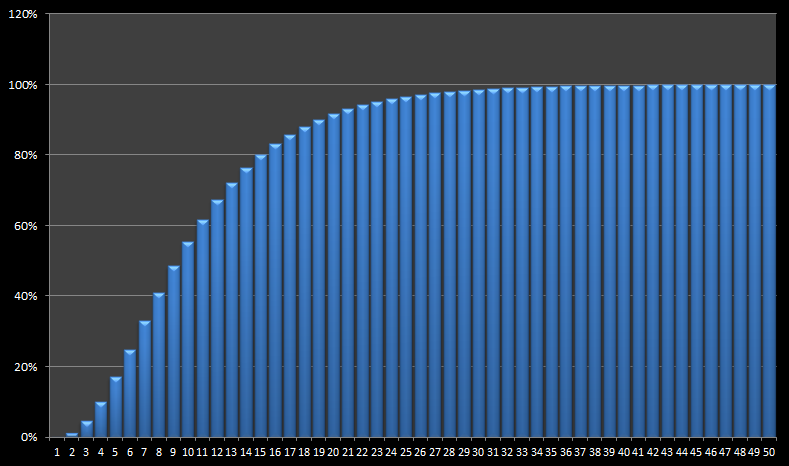The curve asymptotes to 100%, and crosses over 50% by roll #10. To be 95% confident of rolling a Yahtzee you need 23 rolls.

SingletonPairThree of a kindFour of a kindYahtzee
Roll 19.259%69.444%19.290%1.929%0.077%
Roll 20.857%45.010%40.902%11.967%1.263%
Roll 30.079%25.601%45.240%24.476%4.603%
Roll 40.007%14.278%40.914%34.743%10.058%
Roll 50.001%7.937%33.702%41.309%17.051%
Roll 60.000%4.410%26.344%44.337%24.908%
Roll 70.000%2.450%19.928%44.572%33.050%
Roll 80.000%1.361%14.746%42.849%41.044%
Roll 90.000%0.756%10.745%39.898%48.601%
Roll 100.000%0.420%7.742%36.286%55.553%
Roll 110.000%0.233%5.532%32.418%61.817%
Roll 120.000%0.130%3.928%28.567%67.375%
Roll 130.000%0.072%2.776%24.906%72.246%
Roll 140.000%0.040%1.954%21.531%76.474%
Roll 150.000%0.022%1.372%18.488%80.117%
Roll 160.000%0.012%0.961%15.790%83.237%
Roll 170.000%0.007%0.672%13.426%85.895%
Roll 180.000%0.004%0.469%11.375%88.152%
Roll 190.000%0.002%0.327%9.610%90.061%
Roll 200.000%0.001%0.228%8.099%91.671%
Roll 210.000%0.001%0.159%6.813%93.028%
Roll 220.000%0.000%0.111%5.722%94.168%
Roll 230.000%0.000%0.077%4.799%95.124%
Roll 240.000%0.000%0.053%4.020%95.926%
Roll 250.000%0.000%0.037%3.365%96.598%

Often you miss a Yahtzee attempt. What is the breakdown of the probabilities? How likley are you to end up with Four of a kind when shooting for your Yahtzee? This information is easy to obtain by reading the value from the output vector of the Markov Chain for state 4. (The chance that you'll end up with Four of a kind in three rolls is 24.476%, and Three of a kind is 45.240%). The table on the left shows the percentage breakdown for the the first 25 rolls.

Below is the same data presented in graphical format. Notice that after 9 rolls, the Yahtzee becomes the most likely event, and the chance of ending up with a pair or Singleton rapdily falls to noise.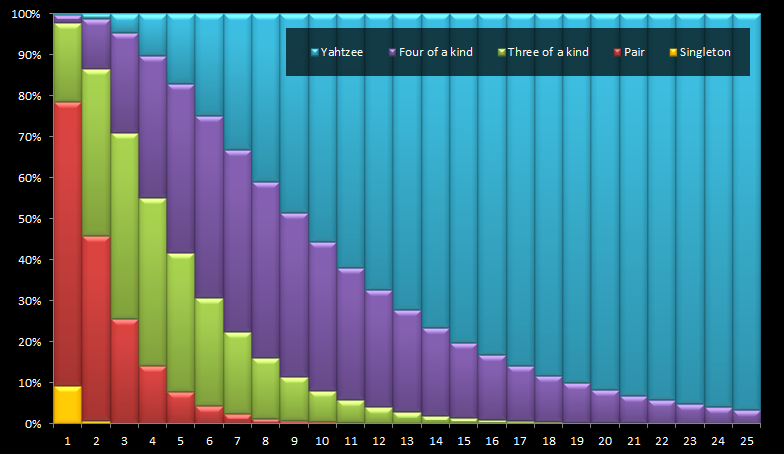### Individual Charts

Here is the same data, but each curve plotted on it's own axis.On the first roll, it's most likely you'll get a pair, but after that the chances of end up with just a pair rapidly decreases. (You'd have to be incredibly unlucky).

You can find a complete list of all the articles here.Click here to receive email alerts on new articles.